# Bias, Variance, and Cross Validation¶

In the last lab, and in homework 2, we alluded to cross-validation with a weak explanation about finding the right hyper-parameters, some of which were regularization parameters. We will have more to say about regularization soon, but lets tackle the reasons we do cross-validation.

The bottom line is: finding the model which has an appropriate mix of bias and variance. We usually want to sit at the point of the tradeoff between the two: be simple but no simpler than necessary.

We do not want a model with too much variance: it would not generalize well. This phenomenon is also called overfitting. There is no point doing prediction if we cant generalize well. At the same time, if we have too much bias in our model, we will systematically underpredict or overpredict values and miss most predictions. This is also known as underfitting.

Cross-Validation provides us a way to find the "hyperparameters" of our model, such that we achieve the balance point.

In :
%matplotlib inline
import numpy as np
import matplotlib.pyplot as plt
import pandas as pd
import brewer2mpl
from matplotlib import rcParams

#colorbrewer2 Dark2 qualitative color table
dark2_cmap = brewer2mpl.get_map('Dark2', 'Qualitative', 7)
dark2_colors = dark2_cmap.mpl_colors

rcParams['figure.figsize'] = (10, 6)
rcParams['figure.dpi'] = 150
rcParams['axes.color_cycle'] = dark2_colors
rcParams['lines.linewidth'] = 2
rcParams['axes.facecolor'] = 'white'
rcParams['font.size'] = 14
rcParams['patch.edgecolor'] = 'white'
rcParams['patch.facecolor'] = dark2_colors
rcParams['font.family'] = 'StixGeneral'

def remove_border(axes=None, top=False, right=False, left=True, bottom=True):
"""
Minimize chartjunk by stripping out unnecesasry plot borders and axis ticks

The top/right/left/bottom keywords toggle whether the corresponding plot border is drawn
"""
ax = axes or plt.gca()
ax.spines['top'].set_visible(top)
ax.spines['right'].set_visible(right)
ax.spines['left'].set_visible(left)
ax.spines['bottom'].set_visible(bottom)

#turn off all ticks
ax.yaxis.set_ticks_position('none')
ax.xaxis.set_ticks_position('none')

#now re-enable visibles
if top:
ax.xaxis.tick_top()
if bottom:
ax.xaxis.tick_bottom()
if left:
ax.yaxis.tick_left()
if right:
ax.yaxis.tick_right()

pd.set_option('display.width', 500)
pd.set_option('display.max_columns', 100)
import warnings
warnings.filterwarnings('ignore', message='Polyfit*')

In :
import random
import copy
def scatter_by(df, scatterx, scattery, by=None, figure=None, axes=None, colorscale=dark2_cmap, labeler={}, mfunc=None, setupfunc=None, mms=8):
cs=copy.deepcopy(colorscale.mpl_colors)
if not figure:
figure=plt.figure(figsize=(8,8))
if not axes:
axes=figure.gca()
x=df[scatterx]
y=df[scattery]
if not by:
col=random.choice(cs)
axes.scatter(x, y, cmap=colorscale, c=col)
if setupfunc:
axeslist=setupfunc(axes, figure)
else:
axeslist=[axes]
if mfunc:
mfunc(axeslist,x,y,color=col, mms=mms)
else:
cs=list(np.linspace(0,1,len(df.groupby(by))))
xlimsd={}
ylimsd={}
xs={}
ys={}
cold={}
for k,g in df.groupby(by):
col=cs.pop()
x=g[scatterx]
y=g[scattery]
xs[k]=x
ys[k]=y
c=colorscale.mpl_colormap(col)
cold[k]=c
axes.scatter(x, y, c=c, label=labeler.get(k,k), s=40, alpha=0.3);
xlimsd[k]=axes.get_xlim()
ylimsd[k]=axes.get_ylim()
xlims=[min([xlimsd[k] for k in xlimsd.keys()]), max([xlimsd[k] for k in xlimsd.keys()])]
ylims=[min([ylimsd[k] for k in ylimsd.keys()]), max([ylimsd[k] for k in ylimsd.keys()])]
axes.set_xlim(xlims)
axes.set_ylim(ylims)
if setupfunc:
axeslist=setupfunc(axes, figure)
else:
axeslist=[axes]
if mfunc:
for k in xs.keys():
mfunc(axeslist,xs[k],ys[k],color=cold[k], mms=mms);
axes.set_xlabel(scatterx);
axes.set_ylabel(scattery);

return axes

def make_rug(axeslist, x, y, color='b', mms=8):
axes=axeslist
zerosx1=np.zeros(len(x))
zerosx2=np.zeros(len(x))
xlims=axes.get_xlim()
ylims=axes.get_ylim()
zerosx1.fill(ylims)
zerosx2.fill(xlims)
axes.plot(x, zerosx1, marker='|', color=color, ms=mms)
axes.plot(zerosx2, y, marker='_', color=color, ms=mms)
axes.set_xlim(xlims)
axes.set_ylim(ylims)
return axes


In any learning problem, or goal is to minimize the prediction error on the test set. This prediction error could be a root mean square error, or a 1-0 loss function, or a log likelyhood, or something else.

## Polynomial regression¶

This part of the lab is partly taken from

http://raw.github.com/jakevdp/sklearn_pycon2013/master/notebooks/09_validation_and_testing.ipynb. Images are taken from Andrew Ng's Coursera course, on which the above notebook is based.

In :
np.random.seed(42)


Consider the model selection problem: what degree of polynomial you want to fit: d. It acts like a hyperparameter, in the sense that it is a second parameter that needs to be fit for. Once you set it, you still have to fit the parameters of your linear or polynomial or elsewise model.

In :
def rmse(p,x,y):
yfit = np.polyval(p, x)
return np.sqrt(np.mean((y - yfit) ** 2))

def generate_curve(x, sigma):
return np.random.normal(10 - 1. / (x + 0.1), sigma)
x = 10 ** np.linspace(-2, 0, 8)
intrinsic_error=1.
y=generate_curve(x, intrinsic_error)
plt.scatter(x,y)

Out:
<matplotlib.collections.PathCollection at 0x105a9cd90>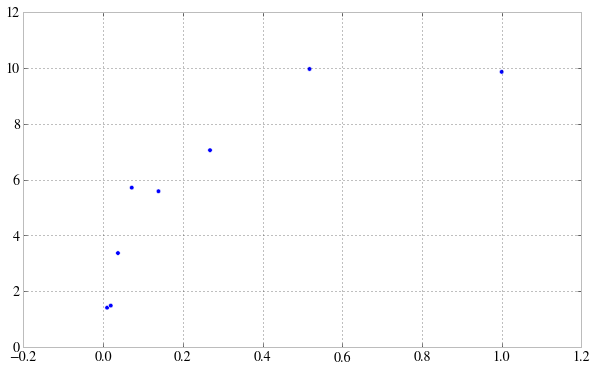A high bias situation is one in which we underfit. Notice how for low d, the rmse on the training set remains high. A high variance situation is one in which we overfit. We want to be just right. As we get to the limit of being able to interpolate the points, the rmse training error goes to nil.

In :
x_new=np.linspace(-0.2, 1.2, 1000)
plt.scatter(x,y, s=50)
f1=np.polyfit(x,y,1)
plt.plot(x_new,np.polyval(f1,x_new))
print "d=1, rmse=",rmse(f1,x,y)
f2=np.polyfit(x,y,2)
plt.plot(x_new,np.polyval(f2,x_new))
print "d=2, rmse=",rmse(f2,x,y)
f4=np.polyfit(x,y,4)
plt.plot(x_new,np.polyval(f4,x_new))
print "d=4, rmse=",rmse(f4,x,y)
f6=np.polyfit(x,y,6)
plt.plot(x_new,np.polyval(f6,x_new))
print "d=6, rmse=",rmse(f6,x,y)
plt.xlim(-0.2, 1.2)
plt.ylim(-1, 12)

d=1, rmse= 1.70524942832
d=2, rmse= 0.89214139212
d=4, rmse= 0.496552676012
d=6, rmse= 0.132980700607

Out:
(-1, 12)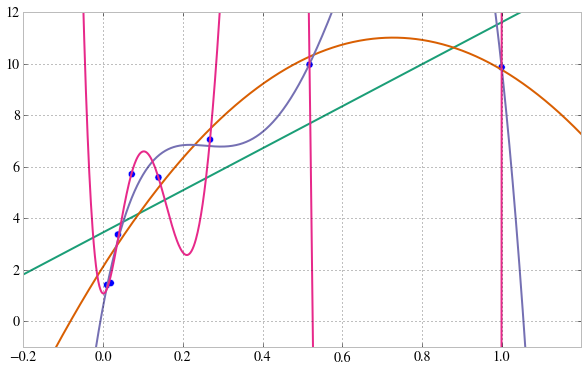The curves start taking on all kinds of wiggles so as to be able to fit themselves in.

### Constructing a data set¶

In :
N = 200
x = np.random.random(N)
y = generate_curve(x, intrinsic_error)
plt.scatter(x,y)

Out:
<matplotlib.collections.PathCollection at 0x105ca6d10>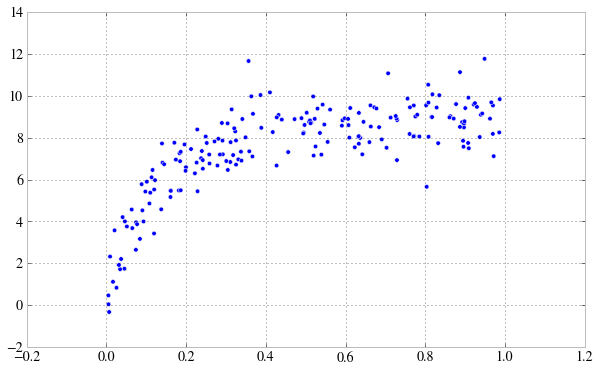In :
from sklearn.cross_validation import train_test_split
xtrain, xtest, ytrain, ytest = train_test_split(x, y, train_size=0.6)
plt.scatter(xtrain, ytrain, color='red')
plt.scatter(xtest, ytest, color='blue')

Out:
<matplotlib.collections.PathCollection at 0x105c837d0>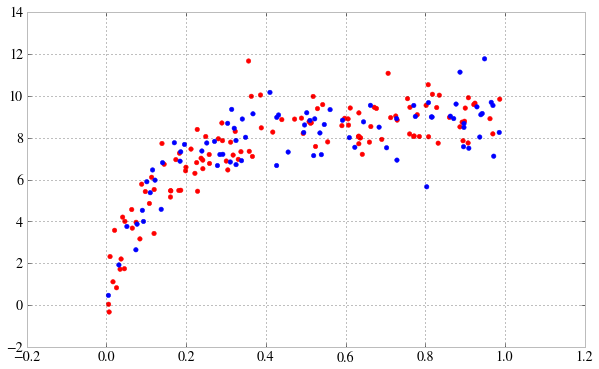In :
ds = np.arange(21)
train_err = np.zeros(len(ds))
test_err = np.zeros(len(ds))

for i, d in enumerate(ds):
p = np.polyfit(xtrain, ytrain, d)

train_err[i] = rmse(p, xtrain, ytrain)
test_err[i] = rmse(p, xtest, ytest)

In :
fig, ax = plt.subplots()

ax.plot(ds, test_err, lw=2, label = 'test error')
ax.plot(ds, train_err, lw=2, label = 'training error')
ax.legend(loc=0)
ax.set_xlabel('degree of fit')
ax.set_ylabel('rms error')

Out:
<matplotlib.text.Text at 0x106729910>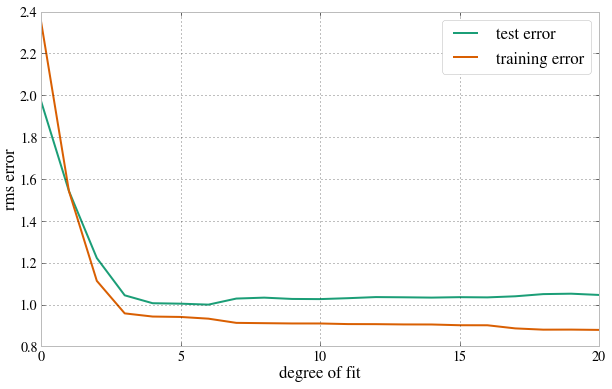How to tell that a hypothesis is overfitting? Its not enough that the training error is low, though thats certainly an indication.

The training error is low but test error is high!

If we plot training error against, say, d, the training error will decrease with increasing d. But for the cross-validation (or for that matter, test error), we'll have an error curve which has a minumum and goes up again.We use the word test and cv interchangeably here, but they really are not, as will be clear soon.

### Learning Curves¶

Here we plot the train vs cv/test error as a function of the size of the training set.

The training set error increases as size of the data set increases. The intuition is that with more samples, you get further away from the interpolation limit. The cross validation error on the otherhand will decrease as training set size increases, as , more data you have better the hypothesis you fit.

High Bias

Now consider the high bias situation. The training error will increase as before, to a point, and then flatten out. (There is only so much you can do to make a straight line fit a quadratic curve). The cv/test error, on the other hand will decrease, but then, it too will flatten out. These will be very close to each other, and after a point, getting more training data will not help!In :
#taken lock stock and barrel from Vanderplas.
def plot_learning_curve(d):
sizes = np.linspace(2, N, 50).astype(int)
train_err = np.zeros(sizes.shape)
crossval_err = np.zeros(sizes.shape)

for i, size in enumerate(sizes):
# Train on only the first size points
p = np.polyfit(xtrain[:size], ytrain[:size], d)

# Validation error is on the *entire* validation set
crossval_err[i] = rmse(p, xtest, ytest)

# Training error is on only the points used for training
train_err[i] = rmse(p, xtrain[:size], ytrain[:size])

fig, ax = plt.subplots()
ax.plot(sizes, crossval_err, lw=2, label='validation error')
ax.plot(sizes, train_err, lw=2, label='training error')
ax.plot([0, N], [intrinsic_error, intrinsic_error], '--k', label='intrinsic error')

ax.set_xlabel('training set size')
ax.set_ylabel('rms error')

ax.legend(loc=0)

ax.set_xlim(0, 99)

ax.set_title('d = %i' % d)

In :
plot_learning_curve(d=1)
plt.ylim(0, 10)

Out:
(0, 10)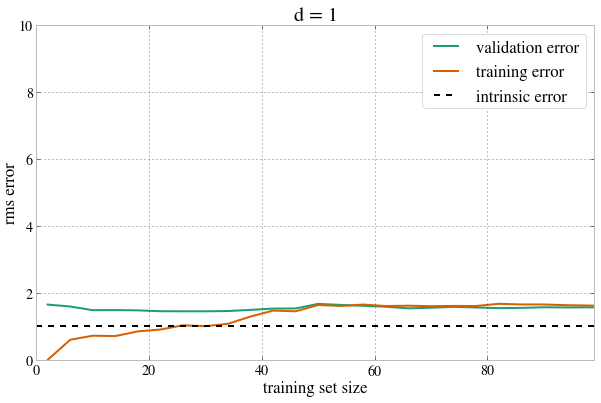At the point of balance the learning curves come together and carry on, close to the intrinsic error.

In :
plot_learning_curve(d=5)
plt.ylim(0, 10)

Out:
(0, 10)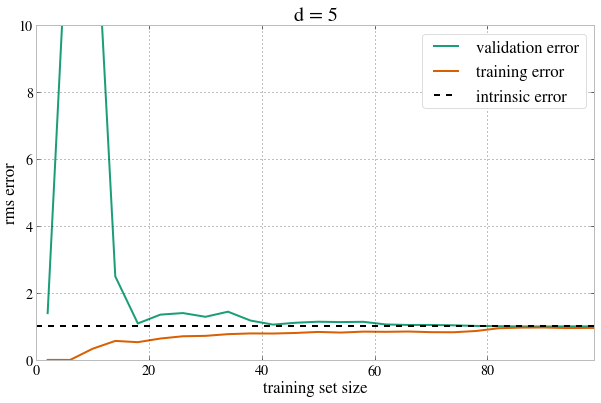Next consider the high variance situation. The training error will start out very low as usual, and go up slowly as even though we add points, we have enough wiggle room to start with, until it runs out and the error keeps increasing. The cv error, will, on the other hand, start out quite high, and remain high. Thus we will have a gap. In this case it will make sense to take more data, as that would drive the cv error down, and the training error up, until they meet.In :
plot_learning_curve(d=20)
plt.ylim(0, 10)

Out:
(0, 10)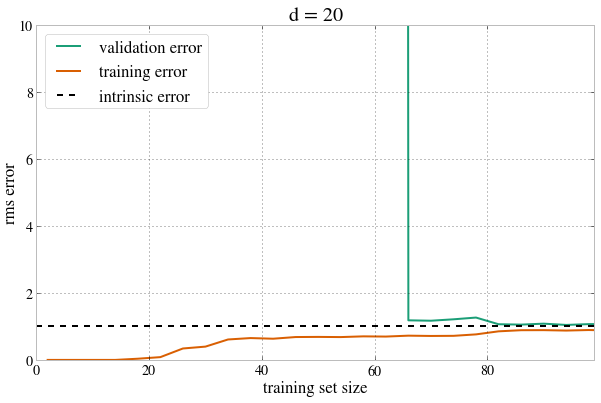### K-Nearest Neighbors¶

In :
df=pd.read_csv("data/olive.csv")

Out:
Unnamed: 0 region area palmitic palmitoleic stearic oleic linoleic linolenic arachidic eicosenoic
0 1.North-Apulia 1 1 1075 75 226 7823 672 36 60 29
1 2.North-Apulia 1 1 1088 73 224 7709 781 31 61 29
2 3.North-Apulia 1 1 911 54 246 8113 549 31 63 29
3 4.North-Apulia 1 1 966 57 240 7952 619 50 78 35
4 5.North-Apulia 1 1 1051 67 259 7771 672 50 80 46
In :
df.rename(columns={df.columns:'areastring'}, inplace=True)
df.areastring=df.areastring.map(lambda x: x.split('.')[-1])
acidlist=['palmitic', 'palmitoleic', 'stearic', 'oleic', 'linoleic', 'linolenic', 'arachidic', 'eicosenoic']
dfsub=df[acidlist].apply(lambda x: x/100.0)
df[acidlist]=dfsub

Out:
areastring region area palmitic palmitoleic stearic oleic linoleic linolenic arachidic eicosenoic
0 North-Apulia 1 1 10.75 0.75 2.26 78.23 6.72 0.36 0.60 0.29
1 North-Apulia 1 1 10.88 0.73 2.24 77.09 7.81 0.31 0.61 0.29
2 North-Apulia 1 1 9.11 0.54 2.46 81.13 5.49 0.31 0.63 0.29
3 North-Apulia 1 1 9.66 0.57 2.40 79.52 6.19 0.50 0.78 0.35
4 North-Apulia 1 1 10.51 0.67 2.59 77.71 6.72 0.50 0.80 0.46
In :
dfsouth = df[df.region==1]
dfsouthns = dfsouth[df.area != 4]

/Users/rahul/anaconda/lib/python2.7/site-packages/pandas/core/frame.py:1943: UserWarning: Boolean Series key will be reindexed to match DataFrame index.
"DataFrame index.", UserWarning)

Out:
areastring region area palmitic palmitoleic stearic oleic linoleic linolenic arachidic eicosenoic
0 North-Apulia 1 1 10.75 0.75 2.26 78.23 6.72 0.36 0.60 0.29
1 North-Apulia 1 1 10.88 0.73 2.24 77.09 7.81 0.31 0.61 0.29
2 North-Apulia 1 1 9.11 0.54 2.46 81.13 5.49 0.31 0.63 0.29
3 North-Apulia 1 1 9.66 0.57 2.40 79.52 6.19 0.50 0.78 0.35
4 North-Apulia 1 1 10.51 0.67 2.59 77.71 6.72 0.50 0.80 0.46
In :
akeys=[1,2,3]
avals=['North-Apulia','Calabria','South-Apulia']
amap={e:e for e in zip(akeys,avals)}
ax=scatter_by(dfsouthns, 'palmitic', 'palmitoleic', by='area', labeler=amap, mfunc=make_rug, mms=20)
ax.grid(False)
ax.legend(loc='upper right');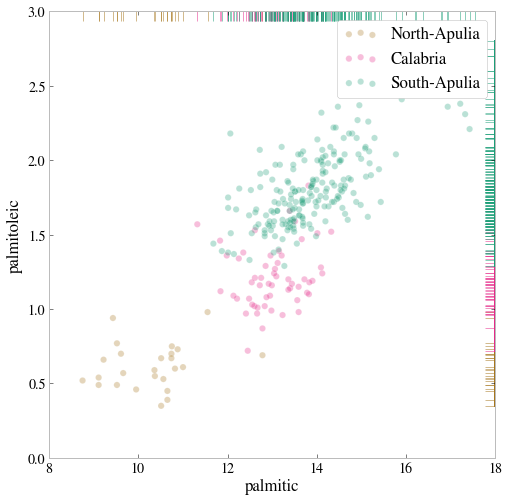In :
from matplotlib.colors import ListedColormap
#cm_bright = ListedColormap(['#FF0000', '#000000','#0000FF'])
#cm = plt.cm.RdBu
cmap_light = ListedColormap(['#FFAAAA', '#AAFFAA', '#AAAAFF'])
cmap_bold = ListedColormap(['#FF0000', '#00FF00', '#0000FF'])

def points_plot(X, Xtr, Xte, ytr, yte, clf, colorscale=cmap_light, cdiscrete=cmap_bold):
h = .02
x_min, x_max = X[:, 0].min() - .5, X[:, 0].max() + .5
y_min, y_max = X[:, 1].min() - .5, X[:, 1].max() + .5
xx, yy = np.meshgrid(np.linspace(x_min, x_max, 50),
np.linspace(y_min, y_max, 50))

plt.figure()
Z = clf.predict(np.c_[xx.ravel(), yy.ravel()])
Z = Z.reshape(xx.shape)
plt.pcolormesh(xx, yy, Z, cmap=cmap_light, alpha=0.2)
plt.scatter(Xtr[:, 0], Xtr[:, 1], c=ytr-1, cmap=cdiscrete, s=50, alpha=0.2,edgecolor="k")
# and testing points
yact=clf.predict(Xte)
print "SCORE", clf.score(Xte, yte)
plt.scatter(Xte[:, 0], Xte[:, 1], c=yte-1, cmap=cdiscrete, alpha=0.5, marker="s", s=35)
plt.xlim(xx.min(), xx.max())
plt.ylim(yy.min(), yy.max())
return ax

In :
from sklearn.neighbors import KNeighborsClassifier
subdf=dfsouthns[['palmitic','palmitoleic']]
subdfstd=(subdf - subdf.mean())/subdf.std()
X=subdfstd.values
y=dfsouthns['area'].values
Xtrain, Xtest, ytrain, ytest = train_test_split(X, y, train_size=0.6)
Xtr=np.concatenate((Xtrain, Xtest))


We do kNN with 20 neighbors. kNN

In :
clf = KNeighborsClassifier(20, warn_on_equidistant=False).fit(Xtrain, ytrain)
points_plot(Xtr, Xtrain, Xtest, ytrain, ytest, clf)

SCORE 0.930434782609

Out:
<matplotlib.axes.AxesSubplot at 0x106821750>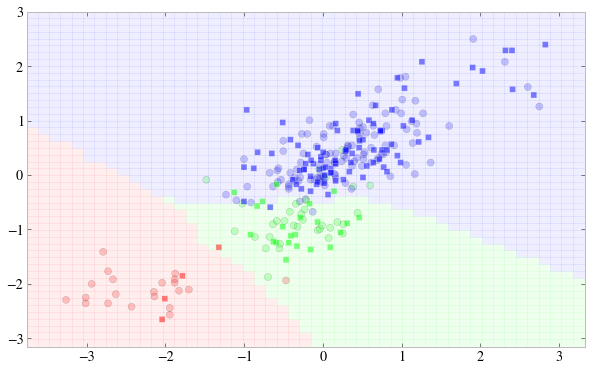What if we decide to get ultra local. We get high variance and Jagged islands.

In :
#your code here
clf = KNeighborsClassifier(1, warn_on_equidistant=False).fit(Xtrain, ytrain)
points_plot(Xtr, Xtrain, Xtest, ytrain, ytest, clf)

SCORE 0.913043478261

Out:
<matplotlib.axes.AxesSubplot at 0x106821750>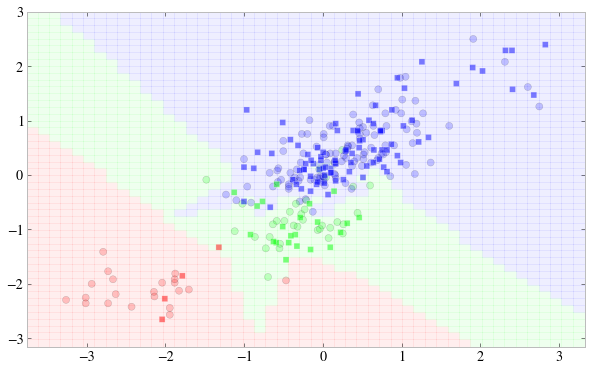You do it for 35 now..see what happens?

In :
#your code here
clf = KNeighborsClassifier(35, warn_on_equidistant=False).fit(Xtrain, ytrain)
points_plot(Xtr, Xtrain, Xtest, ytrain, ytest, clf)

SCORE 0.939130434783

Out:
<matplotlib.axes.AxesSubplot at 0x106821750>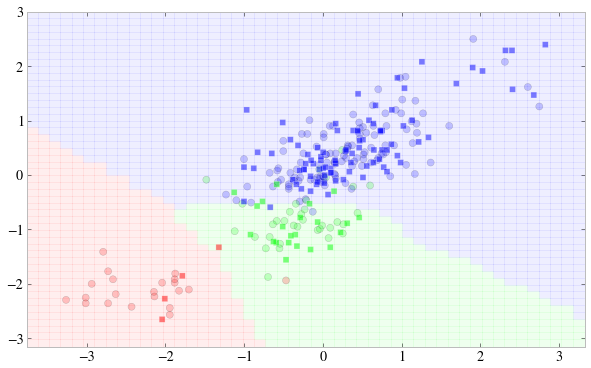We now start splitting data even more in a strange way. Why do we need to do this?

In :
Xcv,Xte,ycv,yte=train_test_split(Xtest, ytest, train_size=0.5)
print ytrain.shape, ycv.shape, yte.shape
trscores=[]
cvscores=[]
ns=np.arange(1,80,1)
ones=np.ones(len(ns))
for n in ns:
clf = KNeighborsClassifier(n, warn_on_equidistant=False).fit(Xtrain, ytrain)
trscores.append(clf.score(Xtrain, ytrain))
cvscores.append(clf.score(Xcv, ycv))
plt.plot(ns, ones-trscores, label="training")
plt.plot(ns, ones-cvscores, label="cv")
plt.legend(loc='upper left');
print clf.score(Xte, yte)

(172,) (57,) (58,)
0.775862068966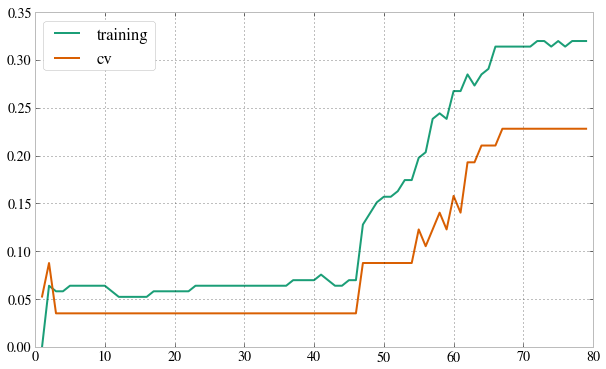This is the same graph we saw earlier, but rversed..k=1 is high variance!

### train/validate/test¶

If you fit hyperparameter by finding the lowest test set error, you can ask how well this model generalize. But this is not likely to be a fair estimate of how well this model generalizes. It is likely to be an optimistic estimate as d is fit to the test set.

So do a 3 way split to train/cv/test and pick hypothesis with lowest cross-validation error. But this might be hard if we didnt have that large a data set.

Practically what we do is to nest our cross-validation inside a grid search. Remember, our hyperparameter fit is also a fit!, and thus the X_cv serves as its "data". Thus we need this nesting and 3 way split. Or you would be testing the hyperparameter fit on its own "training" set.

In :
Xtrain, Xtest, ytrain, ytest = train_test_split(X, y, train_size=0.8)
Xtr=np.concatenate((Xtrain, Xtest))

In :
from sklearn.grid_search import GridSearchCV
from sklearn.metrics import classification_report
parameters = {"n_neighbors": np.arange(1,80,1)}
clf = KNeighborsClassifier(warn_on_equidistant=False)
gs = GridSearchCV(clf, param_grid=parameters, cv=10)
gs.fit(Xtrain, ytrain)
#print gs.grid_scores_
print gs.best_params_, gs.best_score_
y_true, y_pred = ytest, gs.predict(Xtest)
print(classification_report(y_true, y_pred))

{'n_neighbors': 31} 0.94039408867
precision    recall  f1-score   support

1       1.00      1.00      1.00         3
2       1.00      0.70      0.82        10
3       0.94      1.00      0.97        45

avg / total       0.95      0.95      0.94        58


In :
points_plot(Xtr, Xtrain, Xtest, ytrain, ytest, gs)

SCORE 0.948275862069

Out:
<matplotlib.axes.AxesSubplot at 0x106821750>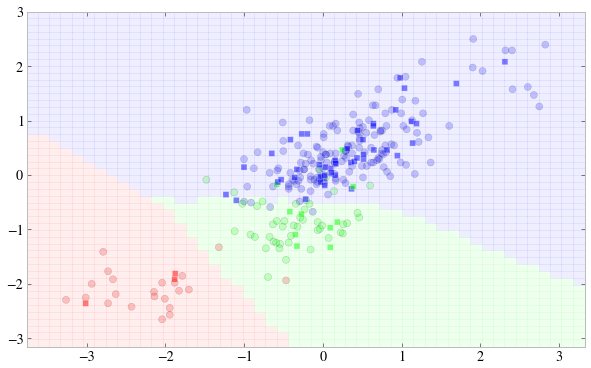We've seen the interplay of bias and variance, and we've seen how hyperparameter, here d and k must be fit by cross-validation, using a nested scheme.

See Chris's notebook for bad consequences that might arise otherwise!

We'd touched on regularization last time, and will revisit regularization next time in the bayesian scheme, and see how regularization parameters control bias and variance.

Finally, we'll put all this together to come up with a strategy for evaluating classifiers and improving our predictions, using this notion of the bias-variance tradeoff, and hyperparameter estimation.